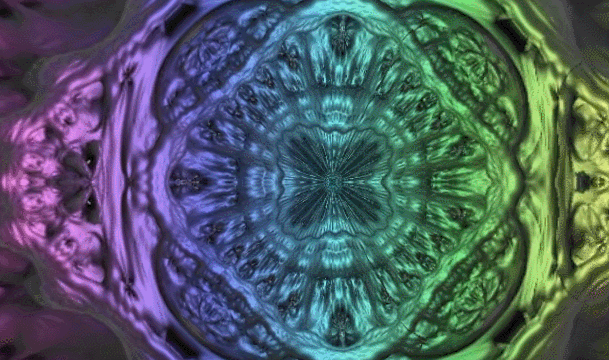# The Mathematics of Rayleigh ScatteringThis post introduces the Mathematics of Rayleigh Scattering, which is the optical phenomenon that causes the sky to appear blue. The equations derived in this tutorial will be translated into shader code in the next tutorial.You can find all the post in this series here:

You can download the Unity package for this tutorial at the bottom of the page.

# The Theory Behind Atmospheric ScatteringThis is the second part of the tutorial on volumetric atmospheric scattering. In this post we will start deriving the equations that govern this complex, yet beautiful optical phenomenon.You can find all the post in this series here:

You can download the Unity package for this tutorial at the bottom of the page.

# Volumetric Atmospheric ScatteringIf you have lived long enough on planet Earth, you might have wondered why the sky is usually blue, yet red at sunset. The optical phenomenon which is (mostly) responsible for that is called Rayleigh scattering. This tutorial will explain how to model atmospheric scattering to reproduce many of the visual effects that planets exhibit. And if you want to render physically accurate visuals for alien planets, this is definitely the tutorial you’ve been looking for.You can find all the post in this series here:

You can download the Unity package for this tutorial at the bottom of the page.

# The Mathematics of Diffraction GratingThis post introduces the mathematics behind the optical phenomenon known as diffraction grating, which is responsible for iridescent reflections in many materials.You can find the complete series here:

A link to download the Unity project used in this series is also provided at the end of the page.

# Understanding Diffraction GratingThe first post in this series, The Nature of Light, introduced the dual nature of light, exhibiting behaviours which are typical of both waves and particles. In this part, we will see how those two aspects are both necessary for iridescence to arise.

You can find the complete series here:

A link to download the Unity project used in this series is also provided at the end of the page.

# Improving the Rainbow – Part 2In the previous part of this tutorial, Improving the Rainbow – Part 1, we have seen different techniques to reproduce the colours of the rainbow procedurally. Solving this problem efficiently will allow us to simulate physically based reflections with a much higher fidelity.

The purpose of this post is to introduce a novel approach that yields better results than any of the previous solutions, without using any branching.You can find the complete series here:

A link to download the Unity project used in this series is also provided at the end of the page.

# Improving the Rainbow – Part 1Our journey to photorealism requires us to understand not only how light works, but also how we perceive colours. How many colours are in the rainbow? And why pink is not one of them? Those are some of the questions that this post will address.You can find the complete series here:

A link to download the Unity project used in this series is also provided at the end of the page.

# The Nature of LightThis is the first part of the tutorial on iridescence. This new series will explore the very nature of light, in order to understand and to replicate how material exhibits colourful reflections. The tutorial is oriented to Unity game developers, although the techniques described can be easily implemented in other languages, including Unreal and WebGL.viYou can find the complete series here:

# Understanding Geographical CoordinatesThis series introduces the concept of trilateration. This technique can be applied to a wide range of problems, from indoor localisation to earthquake detection. This first post provides a general introduction to the concept of geographical coordinates, and how they can be effectively manipulated. The second post in the series, Positioning and Trilateration, will cover the actual techniques used to identify the position of an object given independent distance readings. Most trilateration tutorials require the measures from the sensors to be precise and consistent. The approach here presented, instead, is highly robust and can tolerate inaccurate readings.

# Fractals 101: The Mandelbrot SetThis is the second part of Fractals 101, a series of tutorial dedicated to fractals. This post will investigate two popular fractals: the Mandelbrot set and its 3D cousin, the Mandelbulb.